# Higher-dimensional category

(diff) ← Older revision | Latest revision (diff) | Newer revision → (diff)-category

Letbe a natural number. An-category[a16] consists of sets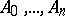, where the elements of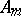are called-arrows and are, for all, equipped with a category structure for whichis the set of objects andis the set of arrows, where the composition is denoted by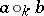(for composable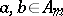), such that, for all, there is a-category (cf. Bicategory) with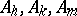, as set of objects, arrows and-arrows, respectively, with vertical composition, and with horizontal composition. The setswith the source and target functions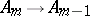form the underlying globular set (or-graph) of. Forand for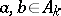with the same-source and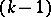-target, there is an-category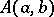whose-arrows (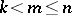) are the-arrows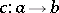of. In particular, for-arrows(also called objects), there is an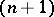-category. This provides the basis of an alternative definition [a17] of-category using recursion and enriched categories [a32] It follows that there is an-category-, whose objects are-categories and whose-arrows are-functors. For infinite, the notion of an-category [a44] is obtained. An-groupoid is an-category such that, for all, each-arrow is invertible with respect to the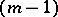-composition (forinfinite,-groupoid is used in [a9] rather than-groupoid, by which they mean something else).

One reason for studying-categories was to use them as coefficient objects for non-Abelian cohomology (cf. Cohomology). This required constructing the nerve of an-category which, in turn, required extending the notion of computad (cf. Bicategory) to-computad, defining free-categories on-computads, and formalising-pasting [a46]; [a22]; [a47]; [a23]; [a41].

Ever since the appearance of bicategories (i.e. weak-categories, cf. Bicategory) in 1967, the prospect of weak-categories () has been contemplated with some trepidation [a37], p. 1261. The need for monoidal bicategories arose in various contexts, especially in the theory of categories enriched in a bicategory [a53], where it was realized that a monoidal structure on the base was needed to extend results of usual enriched category theory [a32]. The general definition of a monoidal bicategory (as the one object case of a tricategory) was not published until [a19]; however, in 1985, the structure of a braiding [a26] was defined on a monoidal (i.e. tensor) categoryand was shown to be exactly what arose when a tensor product (independent of specific axioms) was present on the one-object bicategory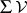. The connection between braidings and the Yang–Baxter equation was soon understood [a52], [a25]. This was followed by a connection between the Zamolodchikov equation and braided monoidal bicategories [a29], [a30] using more explicit descriptions of this last structure. The categorical formulation of tangles in terms of braiding plus adjunction (or duality; cf. also Adjunction theory) was then developed [a18]; [a45]; [a43]. See [a31] for the role this subject plays in the theory of quantum groups.

Not every tricategory is equivalent (in the appropriate sense) to a-category: the interchange law between- and-compositions needs to be weakened from an equality to an invertible coherent-cell; the groupoid case of this had arisen in unpublished work of A. Joyal and M. Tierney on algebraic homotopy-types in the early 1980s; details, together with the connection with loop spaces (cf. Loop space), can be found in [a8]; [a5]. (A different non-globular higher-groupoidal homotopy-type for allwas established in [a35].) Whereas-categories are categories enriched in the category-of-categories with Cartesian product as tensor product, Gray categories (or "semi-strict 3-categories" ) are categories enriched in the monoidal category-where the tensor product is a pseudo-version of that defined in [a20]. The coherence theorem of [a19] states that every tricategory is (tri)equivalent to a Gray category. A basic example of a tricategory is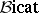whose objects are bicategories, whose arrows are pseudo-functors, whose-arrows are pseudo-natural transformations, and whose-arrows are modifications.

While a simplicial approach to defining weak-categories for allwas suggested in [a46], the first precise definition was that of J. Baez and J. Dolan [a2] (announced at the end of 1995). Other, apparently quite different, definitions by M.A. Batanin [a6] and Z. Tamsamani [a50] were announced in 1996 and by A. Joyal [a24] in 1997. Both the Baez–Dolan and Batanin definitions involve different generalizations of the operads of P. May [a39] as somewhat foreshadowed by T. Trimble, whose operad approach to weak-categories had led to a definition of weak-category (or tetracategory) [a51].

With precise definitions available, the question of their equivalence is paramount. A modified version [a21] of the Baez–Dolan definition together with generalized computad techniques from [a7] are expected to show the equivalence of the Baez–Dolan and Batanin definitions.

The next problem is to find the correct coherence theorem for weak-categories: What are the appropriately stricter structures generalizing Gray categories for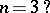Strong candidates seem to be the "teisi" (Welsh for "stacks" ) of [a12], [a13], [a14]. Another problem is to find a precise definition of the weak-category of weak-categories.

The geometry of weak-categories (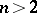) is only at its early stages [a40], [a18], [a33], [a3]; however, there are strong suggestions that this will lead to constructions of invariants for higher-dimensional manifolds and have application to conformal field theory [a10], [a1], [a11], [a36].

The theory of weak-categories, even for, is also in its infancy [a15], [a38]. Reasons for developing this theory, from the computer science viewpoint, are described in [a42]. There are applications to concurrent programming and term-rewriting systems; see [a48], [a49] for references.

How to Cite This Entry:
Higher-dimensional category. Encyclopedia of Mathematics. URL: http://encyclopediaofmath.org/index.php?title=Higher-dimensional_category&oldid=19090
This article was adapted from an original article by Ross Street (originator), which appeared in Encyclopedia of Mathematics - ISBN 1402006098. See original article